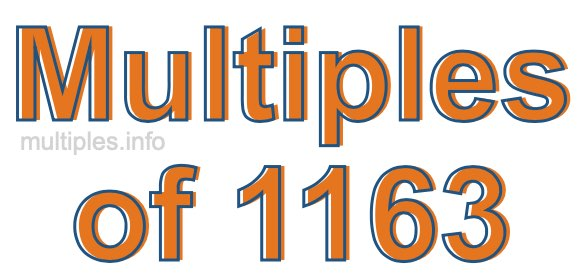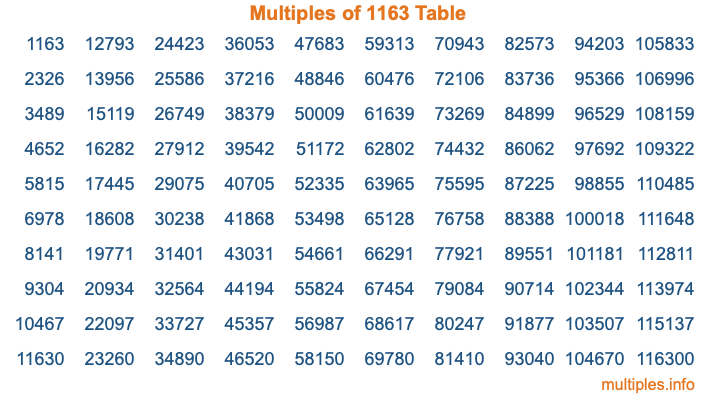Multiples of 1163Welcome to the Multiples of 1163 page. Here we will first teach you everything you will ever need to know about the multiples of 1163, and then give you a study guide summary of everything we taught you to make sure you remember it all. Use this page to look up facts and learn information about the multiples of 1163. This page will make you a multiples of one thousand one hundred sixty-three expert!

Definition of Multiples of 1163
Multiples of 1163 are all the numbers that when divided by 1163 equal an integer. Each of the multiples of 1163 are called a multiple. A multiple of 1163 is created by multiplying 1163 by an integer.

Therefore, to create a list of multiples of 1163, you start with 1 multiplied by 1163, then 2 multiplied by 1163, then 3 multiplied by 1163, and so on for as long as you want. Thus, the list of the first five multiples of 1163 is 1163, 2326, 3489, 4652, and 5815. To see a larger list of multiples of 1163, see the printable image of Multiples of 1163 further down on this page. We also have a category where you can choose any nth multiple of 1163.

Multiples of 1163 Checker
The Multiples of 1163 Checker below checks to see if any number of your choice is a multiple of 1163. In other words, it checks to see if there is any number (integer) that when multiplied by 1163 will equal your number. To do that, we divide your number by 1163. If the the quotient is an integer, then your number is a multiple of 1163.

Is  a multiple of 1163?

Least Common Multiple of 1163 and ...
A Least Common Multiple (LCM) is the lowest multiple that two or more numbers have in common. This is also called the smallest common multiple or lowest common multiple and is useful to know when you are adding our subtracting fractions. Enter one or more numbers below (1163 is already entered) to find the LCM.

Check out our LCM Calculator if you need more details about the Least Common Multiple or if you need the LCM for different numbers for adding and subtraction fractions.

nth Multiple of 1163
As we stated above, 1163 is the first multiple of 1163, 2326 is the second multiple of 1163, 3489 is the third multiple of 1163, and so on. Enter a number below to find the nth multiple of 1163.

th multiple of 1163

Multiples of 1163 vs Factors of 1163
1163 is a multiple of 1163 and a factor of 1163, but that is where the similarities end. All postive multiples of 1163 are 1163 or greater than 1163. All positive factors of 1163 are 1163 or less than 1163.

Below is the beginning list of multiples of 1163 and the factors of 1163 so you can compare:

Multiples of 1163: 1163, 2326, 3489, 4652, 5815, etc.

Factors of 1163: 1, 1163

As you can see, the multiples of 1163 are all the numbers that you can divide by 1163 to get a whole number. The factors of 1163, on the other hand, are all the whole numbers that you can multiply by another whole number to get 1163.

It's also interesting to note that if a number (x) is a factor of 1163, then 1163 will also be a multiple of that number (x).

Multiples of 1163 vs Divisors of 1163
The divisors of 1163 are all the integers that 1163 can be divided by evenly. Below is a list of the divisors of 1163.

Divisors of 1163: 1, 1163

The interesting thing to note here is that if you take any multiple of 1163 and divide it by a divisor of 1163, you will see that the quotient is an integer.

Multiples of 1163 Table
Below is an image of the first 100 multiples of 1163 in a table. The table is in chronological order, column by column. The first column has the first ten multiples of 1163, the second column has the next ten multiples of 1163, and so on.The Multiples of 1163 Table is also referred to as the 1163 Times Table or Times Table of 1163. You are welcome to print out our table for your studies.

Negative Multiples of 1163
Although not often discussed or needed in math, it is worth mentioning that you can make a list of negative multiples of 1163 by multiplying 1163 by -1, then by -2, then by -3, and so on, to get the following list of negative multiples of 1163:

-1163, -2326, -3489, -4652, -5815, etc.

Multiples of 1163 Summary
Below is a summary of important Multiples of 1163 facts that we have discussed on this page. To retain the knowledge on this page, we recommend that you read through the summary and explain to yourself or a study partner why they hold true.

There are an infinite number of multiples of 1163.

A multiple of 1163 divided by 1163 will equal a whole number.

1163 divided by a factor of 1163 equals a divisor of 1163.

The nth multiple of 1163 is n times 1163.

The largest factor of 1163 is equal to the first positive multiple of 1163.

1163 is a multiple of every factor of 1163.

1163 is a multiple of 1163.

A multiple of 1163 divided by a divisor of 1163 equals an integer.

1163 divided by a divisor of 1163 equals a factor of 1163.

Any integer times 1163 will equal a multiple of 1163.

Multiples of a Number
Here you can get the multiples of another number, all with the same attention to detail as we did for multiples of 1163 on this page.

Multiples of
Multiples of 1164
Did you find our page about multiples of one thousand one hundred sixty-three educational? Do you want more knowledge? Check out the multiples of the next number on our list!